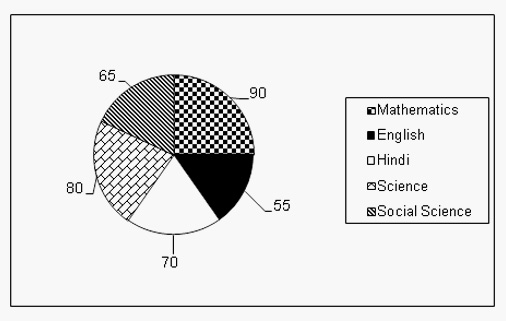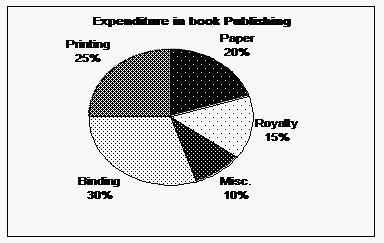# Pie Chart Practice Questions: Level 02

DIRECTIONS for questions 1-5: Refer to the pie chart given below and answer the questions that follow.
The given pie chart shows the marks scored by a student in different subjects- English, Hindi, Mathematics, Science and Social Science in an examination. The values given are in degrees.
Assumption: Total marks obtained in the examination are 900.1. If the total marks were 3000, then marks in Mathematics would be
1. 800
2. 750
3. 850
4. 900
2. The Marks scored in English and Mathematics is less than the marks scored in Science and Hindi by
1. 5%
2. 4.33%
3. 3.33%
4. 6%
3. If the marks scored by the student are 137.5, then the subject is
1. English
2. Hindi
3. Mathematics
4. Science
4. Total marks scored in Social Science and English is
1. 400
2. 350
3. 500
4. 300
5. The difference of marks scored in Social Science and Science is
1. 37.5
2. 40
3. 20
4. 15
DIRECTIONS for questions 6 to 10: The following pie-diagram shows the expenditure incurred in the preparation of a book by a publisher, under various heads.6. What is the difference between the angle of pie- diagram showing the expenditure incurred on binding and printing?
1. 24 deg
2. 48 deg
3. 18 deg
4. 15 deg
7. If the marked price of the book is Rs. 50,what is the cost of printing to publish a single copy of the book ,if the marked price of a book is 25% more than its cost price?
1. Rs.6
2. Rs.10
3. Rs.4.50
4. Rs.6.50
8. By what percentage, expenditure on binding should be reduced so that its expenditure will be equal to paper expenditure?
1. 25%
2. 33.33%
3. 27 2/3%
4. 20%
9. If the sum of two expenditures is represented by 144 degrees in the diagram (if the diagram is made in terms of degrees), these expenditures are
1. Printing & Paper
2. Paper & Binding
3. Printing & Misc
4. Binding & Misc
10. Binding is more than printing charges by
1. 25%
2. 27 2/3%
3. 23.5%
4. 20%